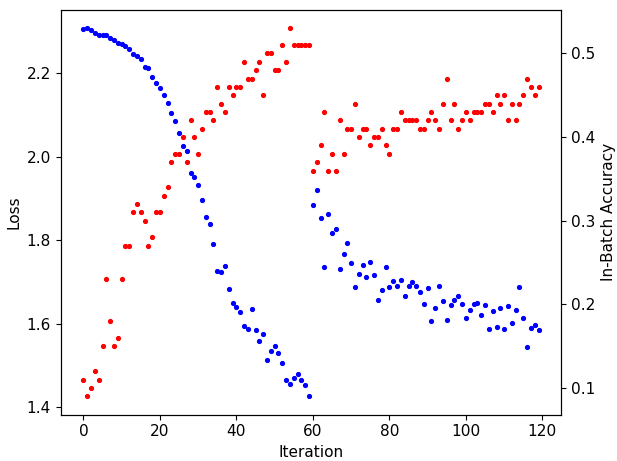# Is it normal that accuracy decreases when advancing to the next epoch?

I’m training a CNN to predict digits using the MNIST database. I’m doing Data Augmentation and for some reason accuracy sharply decreases when advancing to the next epoch (iteration 60 in the image)``````my_transforms = transforms.Compose([
transforms.ToPILImage(),
transforms.RandomCrop((25,25)),
transforms.Resize((28,28)),
transforms.RandomRotation(degrees=45, fill=255),
transforms.RandomVerticalFlip(p=0.1),
transforms.RandomHorizontalFlip(p=0.5),
transforms.ToTensor()
])

dataset = MNISTDataset(transform = my_transforms)
train_loader = DataLoader(dataset = dataset, batch_size = 1000, shuffle=True)

class Net(nn.Module):
def __init__(self):
super().__init__()
self.conv1 = nn.Conv2d(1,10,kernel_size=5)
self.pool = nn.MaxPool2d(kernel_size=2,stride=2)
self.conv2 = nn.Conv2d(10,20,kernel_size=5)
self.fc1 = nn.Linear(20*4*4, 64)
self.fc2 = nn.Linear(64, 10)

def forward(self,x):
x = self.pool(F.relu(self.conv1(x)))
x = self.pool(F.relu(self.conv2(x)))
x = x.view(-1,20*4*4)
x = F.relu(self.fc1(x))
x = F.softmax(self.fc2(x), dim=1)

return x

net = Net()

loss_function=nn.NLLLoss()

EPOCHS=2
iteracion = 0

for epoch in range(EPOCHS):
for data in train_loader:
inputs, labels = data
inputs = inputs.view(-1,1,28,28)

probabilities=net(inputs)

matches=[torch.argmax(i)==int(j) for i,j in zip(probabilities,labels)]

in_batch_acc=matches.count(True)/len(matches)

loss=loss_function(torch.log(probabilities), labels)

print('Loss:', round(float(loss), 3))
print('In-batch acc:', round(in_batch_acc, 2))

iteracion += 1

loss.backward()
optimizer.step()
``````

This is not expected and I cannot reproduce it using your code with some minor modifications:

• use `torchvision.datasets.MNIST` instead of `MNISTDataset`, as it’s undefined
• remove `ToPILImage()`, as `MNIST` already returns `PIL.Images`
• use `F.log_softmax` instead of `torch.log(F.softmax())` for more numerical stability

Here is the output for the switch between epoch 0 and 1:

``````Loss: 1.434
In-batch acc: 0.51
Loss: 1.455
In-batch acc: 0.52
Loss: 1.434
In-batch acc: 0.53
Loss: 1.474
In-batch acc: 0.51
Loss: 1.405
In-batch acc: 0.54
EPOCH 1
Loss: 1.331
In-batch acc: 0.57
Loss: 1.395
In-batch acc: 0.54
Loss: 1.343
In-batch acc: 0.55
Loss: 1.369
In-batch acc: 0.55
Loss: 1.35
In-batch acc: 0.56
Loss: 1.328
In-batch acc: 0.57
Loss: 1.364
``````

@ptrblck instead of using my class MNISTDataset I used torchvision.datasets.MNIST and there’s not accuracy decline when advancing to next epoch. You’re right, so there’s a problem with my class MNISTDataset but I have no clue what could be. Maybe when advancing to next epoch transformations are applied again on the already transformed data? Here is the code of class MNISTDataset:

``````class MNISTDataset(Dataset):
def __init__ (self, transform = None):
xy = np.load('MNIST_train.npy', allow_pickle=True)

self.len = len(xy)

self.transform = transform

x = []
y = []

for i in xy:
x.append(i.reshape(28,28))

y.append(i)

x = np.array(x)/255

y = np.array(y)

self.x = torch.tensor(x, dtype=torch.float)

self.y = torch.tensor(y, dtype=torch.long)

def __getitem__(self, index):
if self.transform:
self.x[index] = self.transform(self.x[index])

return self.x[index], self.y[index]

def __len__(self):
return self.len

my_transforms = transforms.Compose([
transforms.ToPILImage(),
transforms.RandomCrop((25,25)),
transforms.Resize((28,28)),
transforms.RandomRotation(degrees=45, fill=0),
transforms.RandomVerticalFlip(p=0.1),
transforms.RandomHorizontalFlip(p=0.5),
transforms.ToTensor()
])

dataset = MNISTDataset(transform = my_transforms)
train_loader = DataLoader(dataset = dataset, batch_size = 1000, shuffle=True)
``````

Thanks for the code.
Yes, I think you are correct and it seems your custom dataset stores the already transformed samples in these lines of code:

``````    def __getitem__(self, index):
if self.transform:
self.x[index] = self.transform(self.x[index])

return self.x[index], self.y[index]
``````

Avoid the assignment to `self.x` and just return a new tensor via:

``````    def __getitem__(self, index):
if self.transform:
x = self.transform(self.x[index])

return x, self.y[index]
``````